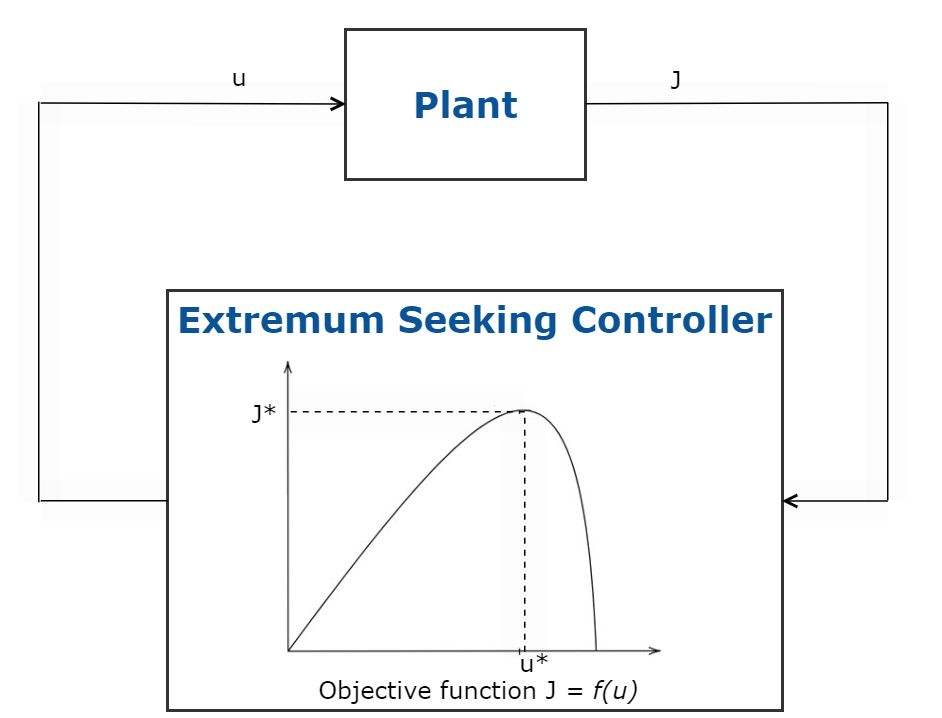# What Is Optimal Control?

Optimal control is a condition of dynamic systems that satisfy design objectives. Optimal control is achieved with control laws that execute following defined optimality criteria. Some widely used optimal control techniques are:

The Linear Quadratic Regulator (LQR) is a full state feedback optimal control law, $$u= -Kx$$, that minimizes a quadratic cost function to regulate the control system.

This cost function depends on system states $$(x)$$ and control inputs $$(u)$$, as shown below.

$$J(u)=\int_{0}^{\infty} (x^T Qx+u^T Ru+2x^T Nu)dt$$

Based on the performance specifications, weighting factors Q, R, and N are set for this optimal control law to define the appropriate balance between system state regulation and the cost of control actuation.

Not all state measurements will be accessible in many optimal control problems. In these cases, states must be estimated using an observer. This is typically done using an observer such as a Kalman filter. A Kalman filter combined with an LQR controller constitutes a Linear Quadratic Gaussian (LQG) controller.

To learn more, check out this MATLAB tech talk (17:23) on LQR control.

## Model Predictive Control

The Model Predictive Control (MPC) is used to minimize a cost function in multi-input multi-output (MIMO) systems that are subject to input and output constraints. This optimal control technique uses a system model to predict future plant outputs. Using the predicted plant outputs, the controller solves an online optimization problem, namely a quadratic program, to determine the optimal adjustments to a manipulable variable that drives the predicted output to the reference. MPC variants include adaptive, gain-scheduled, and nonlinear MPC controllers. The type of MPC controller used depends on the prediction model (linear/nonlinear), constraints (linear/nonlinear), cost function (quadratic/nonquadratic), throughput, and sample time. To learn more, check out this MATLAB Tech Talk (5:59) about MPC variants.

Advances in microprocessor technology and efficient algorithms have increased the adoption of this optimal control method in applications such as automated driving, optimal terrain tracking in aerospace applications, etc.

To learn more, check out this MATLAB Tech Talk series on Model Predictive Control.

## Reinforcement Learning

Reinforcement learning is a machine learning technique in which a computer agent learns optimal behavior through repeated trial-and-error interactions with a dynamic environment. The agent uses observations from the environment to execute a series of actions, with the aim of maximizing the agent’s cumulative reward metric for the task. This learning occurs without human intervention and without explicit programming.

This optimal control method can be used for decision-making problems and as a nonlinear control alternative for applications that use conventional control methods such as automated driving, robotics, scheduling problems, and dynamic calibration of systems.

## Extremum Seeking Control

Extremum seeking is an optimal control technique that automatically adapts control system parameters to maximize an objective function using model-free real-time optimization. This method does not require a system model and can be used for systems where parameters and disturbances slowly change over time. This optimal  control technique is suitable for stable systems that can tolerate noise in control and where only a small number of control system parameters need to be adapted.

Applications of extremum seeking control include adaptive cruise control, maximum power point tracking (MPPT) for solar arrays, and anti-lock braking systems (ABS).Fig 5. Schematic of Extremum Seeking Control.

## H-Infinity synthesis

H-infinity synthesis is an optimal control tool/technique for designing single-input single-output (SISO) or MIMO feedback controllers to achieve robust performance and stability. Compared to classical control techniques such as loop shaping with bode or PID tuning, H-infinity is better suited for multivariable control systems that require cross-coupling between channels.

With H-infinity, the control objectives are formulated in terms of the normalized closed-loop gain. H-infinity synthesis automatically computes a controller that optimizes performance by minimizing this gain. This is useful because many control objectives can be expressed in terms of minimizing gains. This includes objectives such as disturbance rejection, sensitivity to noise, tracking, loop shaping, loop decoupling, and robust stability. Variations of H-infinity synthesis can be used to handle both fixed structure or full-order controllers.

To learn more, check out this MATLAB Tech Talk (13:56) on H-infinity synthesis.

The table below compares the optimal control methods described above:

Optimal control method Is optimization carried out at runtime? (Yes/No) How does the optimization process work for this optimal control process? Can it handle hard constraints?* (Yes/No) Does it use Model-based technique? (Yes/No) What is the throughput? (High/Low)
LQR/LQG No Uses closed-form solution that works with known linear time-invariant systems No Yes High
Implicit MPC (Yes) Using a prediction model, solves an online optimization problem to compute the optimal control actions Yes Yes Low (nonlinear MPC), High (linear MPC)
Explicit MPC (No) Solution to the optimization problem for computing optimal control actions is calculated offline Yes Yes High
Reinforcement Learning Yes** Learns optimal behavior for a task to maximize a reward metric No*** Depends on training algorithm Low (with training), Medium-High (during inference)
Extremum Seeking Control Yes Perturbs and adapts control parameters to maximize an objective function No No High
H-infinity synthesis No Automatically computes a controller that minimizes normalized closed-loop gain No Yes High

* You can impose constraints with the constraint enforcement block. Learn more here.

** With Reinforcement Learning Toolbox™, you can train the agent against a simulated environment. The deployed agent is a trained policy that is not updated at runtime.

*** You can impose action constraints by policy structure and encourage other constraints through reward functions.

See also: What is Reinforcement Learning?, bode plot, frequency response, root locus, PID control, PID tuning